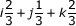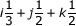# Calculus - Calculus MCQ

11:

Minimum point of the function

is at

 A. x=1 B. x=-1 C. x=0 D. x= 1/√3 Answer Report Discuss Option: A Explanation :  => f'(x)= x2-1  For maximum and minimum value, f'(x) = 0 ∴ x2 -1= 0  x = ± 1 Again f(x) = 2x  At x = 1, f '(x) = 2 > 0  f '(x) = -2 < 0 Hence minimum at x = 1 Click on Discuss to view users comments. ishan said: (1:51am on Friday 30th September 2016) how derivative of fx =x2 -1 while fx= x3/x=x2 Write your comments here:
12:

is equal to

 A. 0 B. ∞ C. 1 D. -1 Answer Report Discuss Option: A Explanation : Click on Discuss to view users comments. Write your comments here:
13:

If f (0) = 2 and f (x) = 1 /  (5-x2), then lower and upper bound of f(1) estimated by the mean value theorem are

 A. 1.9,2.2 B. 2.2,2.25 C. 2.25,2.5 D. None of these Answer Report Discuss Option: B Explanation :Click on Discuss to view users comments. Write your comments here:
14:

The unit normal to the plane 2x +y + 2z = 6 can be expressed in the vector form as

 A. i3 + j2 + k2 B.C.D.Answer Report Discuss Option: B Explanation : Click on Discuss to view users comments. Write your comments here:
15:

Maxima and Minima occur

 A. simultaneously B. once C. alternately D. rarely Answer Report Discuss Option: C Explanation : Click on Discuss to view users comments. Write your comments here: